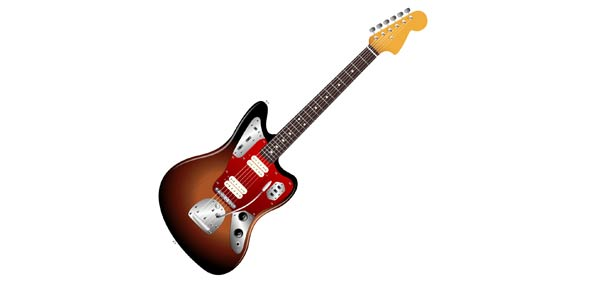# How Much Do You Know About Guitar?

6 Questions | Total Attempts: 422SettingsThis is intended as a evaluation quiz to see where a potential student might fit, or benefit from the program.

• 1.
A standard acoustic guitar has how many strings?
• A.

4

• B.

6

• C.

8

• D.

12

• 2.
The dots on the guitar neck are mainly at the even numbered frets?
• A.

True

• B.

False

• 3.
What is the significance of the double dots?
• A.

It's where the next string starts.

• B.

It's middle C.

• C.

It's an octave.

• D.

It's where the neck ends.

• 4.
Name the open strings on the guitar, from thickest to thinnest...
• A.

E, G, A, D, E, B

• B.

E, B, G, D, A, E

• C.

E, A, G, D, B, E

• D.

E, A, D, G, B, E

• E.

G, D, A, E, B, E

• 5.
Octaves always have the same note name?
• A.

True

• B.

False

• 6.
Show which notes are located a whole step apart from each other...
• A.

B - C

• B.

D - E

• C.

G - A

• D.

F - G#

• E.

Bb - C

• F.

Eb - F

• G.

A# - Bb

Related TopicsBack to top